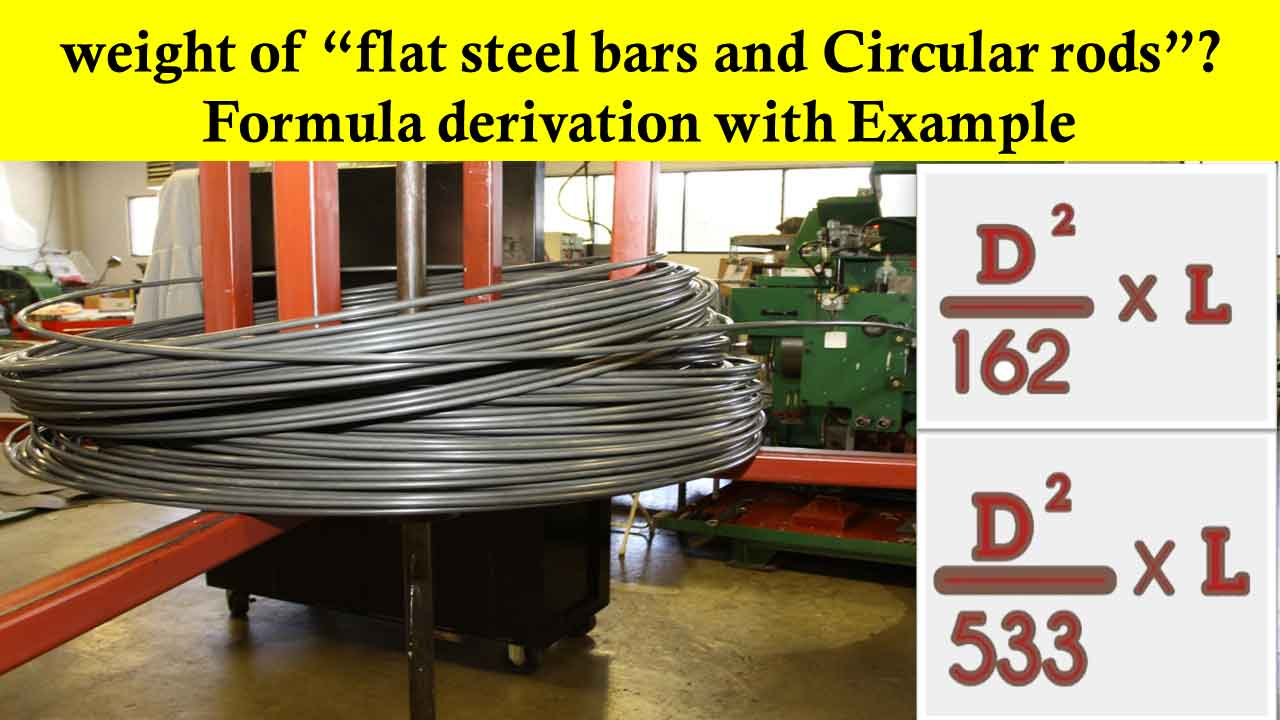# How to calculate the weight of steel flat bars and Circular rods?

Why we have to calculate the weight of steel and why we have to derive the weight of steel bars formula?

For this you need to understand how estimation works, for every project the steel is represented in length as meter, foot, etc,… and diameter of the rod in mm, for example, we estimate steel as 10 meters of 8mm diameter bar or 10 foot of 8mm bar, etc…

This notation looks perfect right! But here comes the big problem, the manufacturers and suppliers will not understand if we say in meters, so what we have to say?

They will measure the steel in kg or quintal or ton to make it easier. This creates a need to calculate the steel in kg or quintal or ton.

So let us discuss how to calculate the weight of steel bars. Before we get into the derivation of the formulae, there are some important terms we have to know;

## What is density or unit weight of steel?

The density of steel varies based on the alloying constituents but usually ranges between 7,750 and 8,050 kg/m3.

Here, the density of steel is taken as 7850kg/m3   for all the calculations of unit weights.

Few Important Articles:

## Weight of steel bars Formula:

Circular and Flat steel reinforcement are the popular types of steel bars used for construction, But most of the time we prefer circular steel rods. so in this article, we will try to derive both the formulas and also we will find the unit weight of some steel rods.

## Unit Weight of flat Steel Bars

The density of mild steel is 7850kg/m3. An MS (mild steel) flat bar is specified by its width and thickness in millimeters (mm). If so, the weight of the steel bar is given by:

Weight of steel bar = Volume of steel x Density of steel

Weight of steel Flat bar = (Length x width x thickness) x 7850

Example 1: Determine the unit weight of MS Flat bar of width 40mm and Thickness of 20mm.

• Weight of steel bar = Volume of steel Bar x Density of steel
• Weight of steel bar = (Length x width x thickness) x 7850
• Unit Weight of steel bar = (1 x .040 x .020) x 7850 = 6.28kg/m

## Unit Weight of Steel bars formula;

Here, the density of steel is taken as 7850kg/m3. An MS (mild steel) round bars are specified by their diameters in millimeters(mm). If so, the weight of the steel bar is given by:

Weight of steel bar = Volume of steel x Density of steel

Weight of steel rod = (Cross sectional area of steel rod x Length) x 7850kg/m3

= (3.14 x (D/4) x L) x 7850kg/m3

= (D2 x 6162.25L)/1000000

= D2 L x 0.006162 = DL / 162.28

• Weight of steel bars formula = [ DL / 162.28] in Kg and Length ‘L’ in m
• Weight of steel bars formula = [ DL / 533] in Kg and Length ‘L’ in foot

To calculate the unit weight, length “L’ becomes unity.

Example 2: Determine the unit weight of 10mm diameter steel bars.

• The formula is: Weight of Steel Bar = [DL / 162.28]
• Hence, the weight of: 10mm dia bar = (10x10x1)/162.28 = 0.616kg

## Unit weight of different diameter bars?

If we put 1 meter in the formulae we will get the unit weight of steel. If we multiply this unit weight with the length of the bar, we will get the total weight of steel bars required for the construction.

## Conclusion

• From the weight of steel bars formula, we calculate the unit weight of the bar either in meter or foot, and we have to multiply unit weight with the length of the bar to get the total weight of the rod.
• In this way, we will find the weight of the steel required for the construction, but we have to note one important point, there may be some variations when we compare these calculated values with the actual steel quantity after completing the construction.

If you are facing any issues with the calculations, please comment below! or try to contact us on Instagram @civilogy### K Vinay Goud

My name is k.vinay. I am a civil engineer from India. I know there were many people who struggle for information during graduation or after seeking their job. I have started this website for providing basic knowledge and New technologies which were implementing all over the world. we will definitely provide valuable information for you all. stay connected and Follow us on other social media platforms. Thank you !